﻿ Dirichlet Average of Generalized Miller-Ross Function and Fractional Derivative

### Dirichlet Average of Generalized Miller-Ross Function and Fractional Derivative

Mohd. Farman Ali, Manoj Sharma, Lakshmi Narayan Mishra, Vishnu N. MishraOPEN ACCESSPEER-REVIEWED

## Dirichlet Average of Generalized Miller-Ross Function and Fractional Derivative

Mohd. Farman Ali1, Manoj Sharma2, Lakshmi Narayan Mishra3, 4, Vishnu N. Mishra5,1School of Mathematics and Allied Sciences, Jiwaji University, Gwalior

2Department of Mathematics RJIT, BSF Academy, Tekanpur, Gwalior

3Department of Mathematics, National Institute of Technology, Silchar - 788 010, District - Cachar (Assam), India

4L. 1627 Awadh Puri Colony Beniganj, Phase – III, Opposite – Industrial Training Institute (I.T.I.), Faizabad - 224 001 (Uttar Pradesh), India

5Applied Mathematics and Humanities Department, Sardar Vallabhbhai National Institute of Technology, Ichchhanath Mahadev Dumas Road, Surat – 395 007 (Gujarat), India

### Abstract

The object of the present paper is to establish the results of single Dirichlet average of Generalized Miller-Ross Function, using Riemann-Liouville Fractional Integral. The Generalized Miller-Ross Function can be measured as a Dirichlet average and connected with fractional calculus. In this paper the solution comes in compact form of single Dirichlet average of Generalized Miller-Ross Function. The special cases of our results are same as earlier obtained by Saxena et al. , for single Dirichlet average of Generalized Miller-Ross Function.

• Ali, Mohd. Farman, et al. "Dirichlet Average of Generalized Miller-Ross Function and Fractional Derivative." Turkish Journal of Analysis and Number Theory 3.1 (2015): 30-32.
• Ali, M. F. , Sharma, M. , Mishra, L. N. , & Mishra, V. N. (2015). Dirichlet Average of Generalized Miller-Ross Function and Fractional Derivative. Turkish Journal of Analysis and Number Theory, 3(1), 30-32.
• Ali, Mohd. Farman, Manoj Sharma, Lakshmi Narayan Mishra, and Vishnu N. Mishra. "Dirichlet Average of Generalized Miller-Ross Function and Fractional Derivative." Turkish Journal of Analysis and Number Theory 3, no. 1 (2015): 30-32.

 Import into BibTeX Import into EndNote Import into RefMan Import into RefWorks

### 1. Introduction

Carlson [1-5] has defined Dirichlet average of functions which represents certain type of integral average with respect to Dirichlet measure. He showed that various important special functions can be derived as Dirichlet averages for the ordinary simple functions like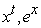etc. He has also pointed out  that the hidden symmetry of all special functions which provided their various transformations can be obtained by averaging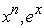etc. Thus he established a unique process towards the unification of special functions by averaging a limited number of ordinary functions. Almost all known special functions and their well known properties have been derived by this process.

Recently, Gupta and Agarwal [9, 10] found that averaging process is not altogether new but directly connected with the old theory of fractional derivative. Carlson overlooked this connection whereas he has applied fractional derivative in so many cases during his entire work. Deora and Banerji  have found the double Dirichlet average of ex by using fractional derivatives and they have also found the Triple Dirichlet Average of xt by using fractional derivatives .

In the present paper the Dirichlet average of Generalized Miller-Ross Function has been obtained.

### 2. Definitions

Some definitions which are necessary in the preparation of this paper.

2.1. Standard Simplex in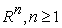:

Denote the standard simplex in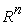,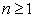by [, p. 62].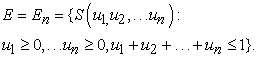2.2. Dirichlet Measure

Let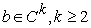and let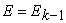be the standard simplex in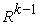The complex measure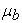is defined by E .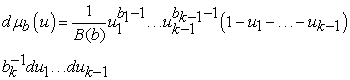known as Dirichlet measure.

Here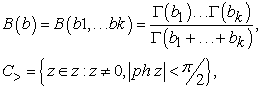open right half plane and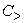k is the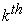Cartesian power of.

2.3. Dirichlet Average[, p. 75]

Let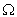be the convex set in, let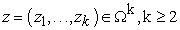and let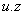be a convex combination of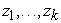. Let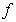be a measureable function onand letbe a Dirichlet measure on the standard simplex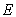in.Define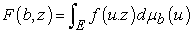(2.3)

F is the Dirichlet measure ofwith variables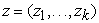and parameters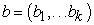.

Here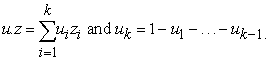If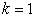, define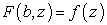2.4. Generalized Miller-Ross Function

This function is introduced by the author as follows:(2.4)

Here,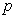upper parameters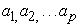and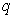lower parameters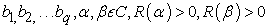and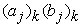are pochammer symbols. The function (3.6.1.1) is defined when none of the denominator parameters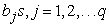is a negative integer or zero. If any parameter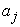is negative then the function (2.4) terminates into a polynomial in x. By using ratio test, it is evident that function (2.4) is convergent for all x, when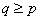, it is convergent for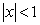when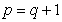, divergent when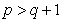. In some cases the series is convergent for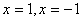. Let us consider take,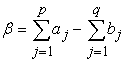when, the series is absolutely convergent for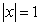if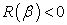, convergent for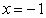if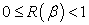and divergent forif 1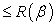which is a special case of Wright function.

2.5. Fractional Derivative [, p. 181]

The theory of fractional derivative with respect to an arbitrary function has been used by Erdelyi . The general definition for the fractional derivative of order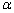found in the literature on the “Riemann-Liouville integral” is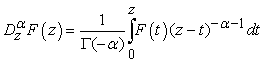(2.5)

where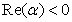and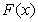is the form of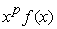, where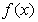is analytic at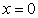### 3. Equivalence

In this section we shall prove the equivalence of single Dirichlet average of Generalized Miller-Ross Function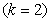with the fractional derivative i.e.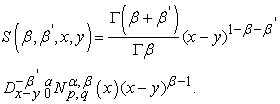(3.1)

Proof: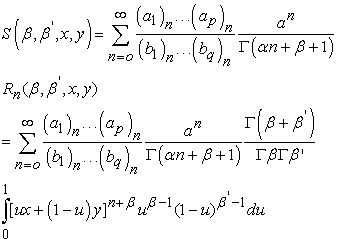Putting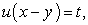we haveOn changing the order of integration and summation, we have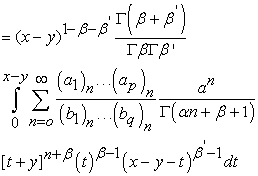Or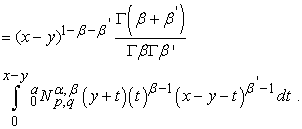Hence by the definition of fractional derivative, we get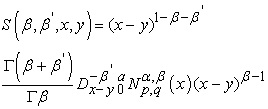This completes the analysis.

### 4. Particular Cases

If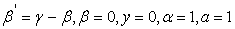and no upper and lower parameter in (3.1) then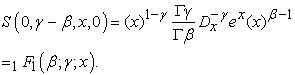(4.1)

This confluent hyper geometric function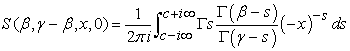Then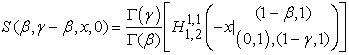(ii) If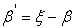and from (4.1), then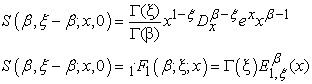where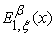be the generalization of Mittag-Leffler function .

### 5. Applications

Dirichlet average is average given by Dirichlet. The Dirichlet average of elementary function like power function, exponential function etc is given by many notable mathematician, Actually, We have convert the elementary function into the summation form after that taking Dirichlet average of those function, using fractional integral and get new results. These results will be used in future by mathematician and scientist. Thus we have find a connection Dirichlet average of a function and fractional integral.

### Acknowledgements

The authors express their thanks to the anonymous learned referee(s) and the editor for their constructive comments, valuable suggestions, which resulted in the subsequent improvement of this research article. The authors are grateful to all editorial board members and reviewers of esteemed journal i.e. Turkish Journal of Applied Analysis and Number Theory (TJANT). All the authors conceived of the study and participated in its design and coordination. All authors drafted the manuscript, participated in the sequence alignment. All the authors read and approved the final version of manuscript. The authors declare that there is no conflict of interests regarding the publication of this research article.

### References

  Carlson, B.C., Special Function of Applied Mathematics, Academic Press, New York, 1977.In article  Carlson, B.C., Appell’s function F4 as a double average, SIAM J.Math. Anal. 6 (1975), 960-965.In article CrossRef  Carlson, B.C., Hidden symmetries of special functions, SIAM Rev. 12 (1970), 332-345.In article CrossRef  Carlson, B.C., Dirichlet averages of x t log x, SIAM J.Math. Anal. 18(2) (1987), 550-565.In article CrossRef  Carlson, B.C., A connection between elementary functions and higher transcendental functions, SIAM J. Appl. Math. 17 (1969), 116-140.In article CrossRef  Deora, Y. and Banerji, P.K., Double Dirichlet average of ex using fractional derivatives, J. Fractional Calculus 3 (1993), 81-86.In article  Deora, Y. and Banerji, P.K., Double Dirichlet average and fractional derivatives, Rev.Tec.Ing.Univ. Zulia 16 (2) (1993), 157-161.In article  Erdelyi, A., Magnus, W., Oberhettinger, F. and Tricomi , F.G., Tables of Integral Transforms, Vol. 2 McGraw-Hill, New York, 1954.In article PubMed  Gupta,S.C. and Agrawal, B.M., Dirichlet average and fractional derivatives, J. Indian Acad.Math. 12(1) (1990), 103-115.In article  Gupta,S.C. and Agrawal, Double Dirichlet average of ex using fractional derivatives, Ganita Sandesh 5 (1) (1991),47-52.In article  Mathai, A.M. and Saxena,R.K., The H-Function with Applications in Stastistics and other Disciplines, Wiley Halsted, New York, 1978.In article  Saxena,R.K., Mathai,A.M and Haubold, H.J., Unified fractional kinetic equation and a fractional diffusion equation, J. Astrophysics and Space Science 209 (2004) , 299-310.In article CrossRef  Sharma, Manoj and Jain, Renu, Dirichlet Average and Fractional Derivatie, J. Indian Acad. Math. Vol. 12, No. 1(1990).In article